# CorrGAN: A GAN for sampling correlation matrices (Part I)

In a previous blog, we exposed how to sample uniformly over the space of correlation matrices. In practice, we may want to sample from a subset of the space of correlation matrices (also called elliptope), which is not clearly defined, but implicitely defined by empirical observations. Generative adversarial networks (GANs) may be a good option to sample from this ‘not well specified subspace’. For example, GANs have been found successful for sampling from the ‘human faces’ subspace of ‘natural images’ subspace of all possible colored images (matrices/tensors) (cf. StyleGAN). The ‘human faces’ and ‘natural images’ subspaces are not formally defined, we only have an understanding of them through many examples.

Before trying to sample from any ‘complex’ subset of the N-dimensional elliptope, let’s focus on the full 3-dimensional elliptope (which corresponds to the set of 3x3 correlation matrices). At least,

1. we know how to sample (uniformly) perfectly from the elliptope so that we can compare with the samples generated by the GAN,
2. we can visualize the results in 3D.

Below some code that allows to sample nearly correctly from the 3D elliptope. Notice that a few points are living outside the hull. They correspond to 3x3 matrices which are not positive semidefinite (hence not proper correlation matrices). Notice that, here, we generate only 3 coefficients since out of the 3x3=9 coefficient, only n(n-1)/2 = 3 matters (3 being forced to 1 (diagonal), 3 are defined by the symmetry). We could ask the GAN to learn the diagonal property (=1) and the symmetry property (C = C^T), but that would make the problem uselessly harder.

TL;DR (Left) Generated samples from vanilla GAN (in orange); (Right) Generated samples from Wasserstein GAN (in orange). Something went wrong… What?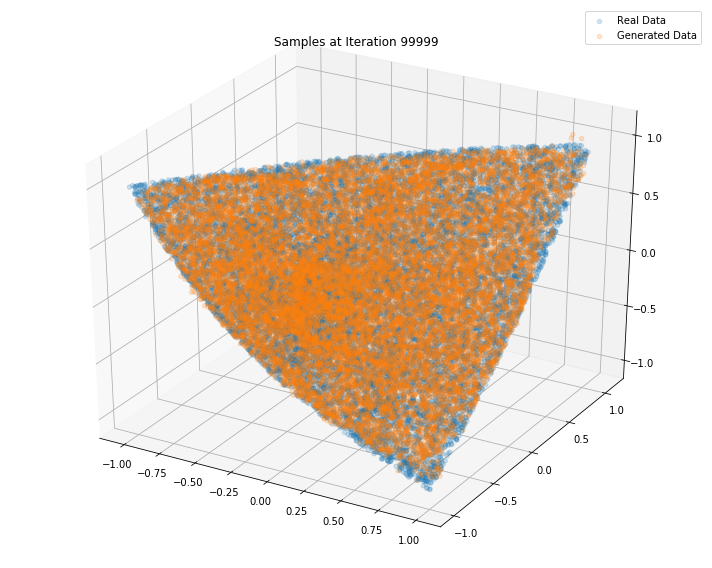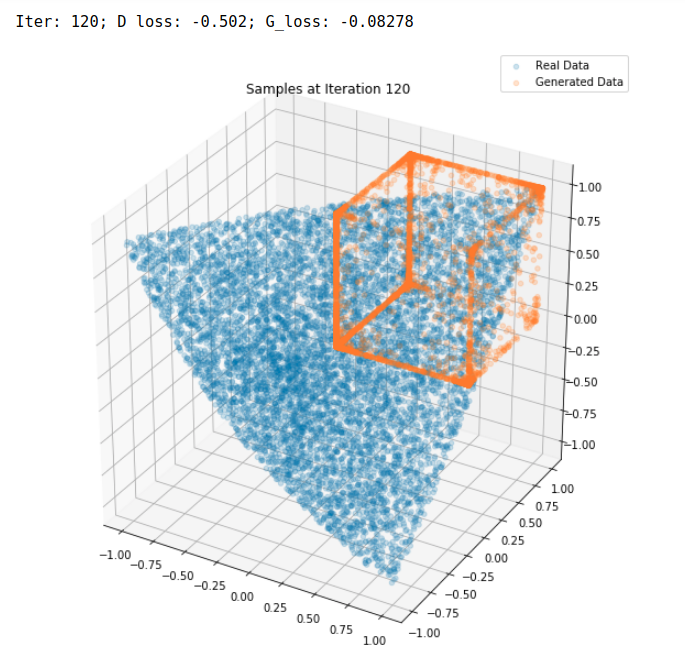``````import numpy as np
from numpy.random import beta
from numpy.random import randn
from scipy.linalg import sqrtm
from numpy.random import seed

seed(42)

def sample_unif_correlmat(dimension):
d = dimension + 1

prev_corr = np.matrix(np.ones(1))
for k in range(2, d):
# sample y = r^2 from a beta distribution with alpha_1 = (k-1)/2 and alpha_2 = (d-k)/2
y = beta((k - 1) / 2, (d - k) / 2)
r = np.sqrt(y)

# sample a unit vector theta uniformly from the unit ball surface B^(k-1)
v = randn(k-1)
theta = v / np.linalg.norm(v)

# set w = r theta
w = np.dot(r, theta)

# set q = prev_corr**(1/2) w
q = np.dot(sqrtm(prev_corr), w)

next_corr = np.zeros((k, k))
next_corr[:(k-1), :(k-1)] = prev_corr
next_corr[k-1, k-1] = 1
next_corr[k-1, :(k-1)] = q
next_corr[:(k-1), k-1] = q

prev_corr = next_corr

return next_corr
``````
``````sample_unif_correlmat(3)
``````
``````array([[ 1.        ,  0.36739638,  0.1083456 ],
[ 0.36739638,  1.        , -0.05167306],
[ 0.1083456 , -0.05167306,  1.        ]])
``````
``````def sample_data(n=10000):
data = []
for i in range(n):
m = sample_unif_correlmat(3)
data.append([m[0, 1], m[0, 2], m[1, 2]])

return np.array(data)
``````
``````from mpl_toolkits.mplot3d import Axes3D
import matplotlib.pyplot as plt

fig = plt.figure(figsize=(10, 8))

xs = []
ys = []
zs = []
d = sample_data()
for datum in d:
xs.append(datum)
ys.append(datum)
zs.append(datum)

ax.scatter(xs, ys, zs, alpha=0.2)

ax.set_xlabel('\$\\rho_{12}\$')
ax.set_ylabel('\$\\rho_{13}\$')
ax.set_zlabel('\$\\rho_{23}\$')

plt.show()
``````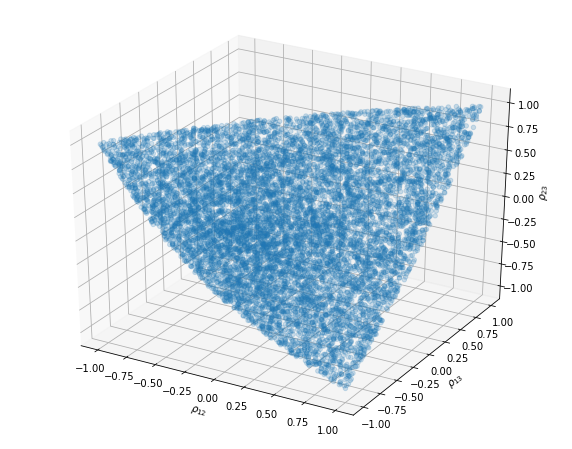``````import tensorflow as tf
import keras
``````
``````Using TensorFlow backend.
``````
``````def generator(Z, hsize=[64, 64, 16], reuse=False):
with tf.variable_scope("GAN/Generator", reuse=reuse):
h1 = tf.layers.dense(Z, hsize, activation=tf.nn.leaky_relu)
h2 = tf.layers.dense(h1, hsize, activation=tf.nn.leaky_relu)
h3 = tf.layers.dense(h2, hsize, activation=tf.nn.leaky_relu)
out = tf.layers.dense(h3, 3)

return out
``````
``````def discriminator(X, hsize=[64, 64, 16], reuse=False):
with tf.variable_scope("GAN/Discriminator", reuse=reuse):
h1 = tf.layers.dense(X, hsize, activation=tf.nn.leaky_relu)
h2 = tf.layers.dense(h1, hsize, activation=tf.nn.leaky_relu)
h3 = tf.layers.dense(h2, hsize, activation=tf.nn.leaky_relu)
h4 = tf.layers.dense(h3, 3)
out = tf.layers.dense(h4, 1)

return out, h4
``````
``````X = tf.placeholder(tf.float32, [None, 3])
Z = tf.placeholder(tf.float32, [None, 3])
``````
``````G_sample = generator(Z)
r_logits, r_rep = discriminator(X)
f_logits, g_rep = discriminator(G_sample, reuse=True)
``````
``````disc_loss = tf.reduce_mean(
tf.nn.sigmoid_cross_entropy_with_logits(
logits=r_logits, labels=tf.ones_like(r_logits))
+ tf.nn.sigmoid_cross_entropy_with_logits(
logits=f_logits, labels=tf.zeros_like(f_logits)))

gen_loss = tf.reduce_mean(
tf.nn.sigmoid_cross_entropy_with_logits(
logits=f_logits, labels=tf.ones_like(f_logits)))
``````
``````gen_vars = tf.get_collection(tf.GraphKeys.GLOBAL_VARIABLES, scope="GAN/Generator")
disc_vars = tf.get_collection(tf.GraphKeys.GLOBAL_VARIABLES, scope="GAN/Discriminator")

gen_step = tf.train.RMSPropOptimizer(
learning_rate=0.0001).minimize(gen_loss, var_list=gen_vars)
disc_step = tf.train.RMSPropOptimizer(
learning_rate=0.0001).minimize(disc_loss, var_list=disc_vars)
``````
``````sess = tf.Session()
tf.global_variables_initializer().run(session=sess)

batch_size = 2**8
nd_steps = 5
ng_steps = 5
``````
``````def sample_Z(m, n):
return np.random.uniform(-1., 1., size=[m, n])

n_dots = 2**12
x_plot = sample_data(n=n_dots)
Z_plot = sample_Z(n_dots, 3)
``````
``````for i in range(100000):
X_batch = sample_data(n=batch_size)
Z_batch = sample_Z(batch_size, 3)

for _ in range(nd_steps):
_, dloss = sess.run([disc_step, disc_loss], feed_dict={X: X_batch, Z: Z_batch})
rrep_dstep, grep_dstep = sess.run([r_rep, g_rep], feed_dict={X: X_batch, Z: Z_batch})

for _ in range(ng_steps):
_, gloss = sess.run([gen_step, gen_loss], feed_dict={Z: Z_batch})

rrep_gstep, grep_gstep = sess.run([r_rep, g_rep], feed_dict={X: X_batch, Z: Z_batch})

if (i <= 1000 and i % 100 == 0) or (i % 10000 == 0):
print("Iterations: %d\t Discriminator loss: %.4f\t Generator loss: %.4f"%(i, dloss, gloss))

fig = plt.figure(figsize=(10, 8))
g_plot = sess.run(G_sample, feed_dict={Z: Z_plot})
ax.scatter(x_plot[:, 0], x_plot[:, 1], x_plot[:, 2], alpha=0.2)
ax.scatter(g_plot[:, 0], g_plot[:, 1], g_plot[:, 2], alpha=0.2)

plt.legend(["Real Data", "Generated Data"])
plt.title('Samples at Iteration %d' % i)
plt.tight_layout()
plt.show()
plt.close()
``````
``````Iterations: 0	 Discriminator loss: 1.3821	 Generator loss: 0.6854
``````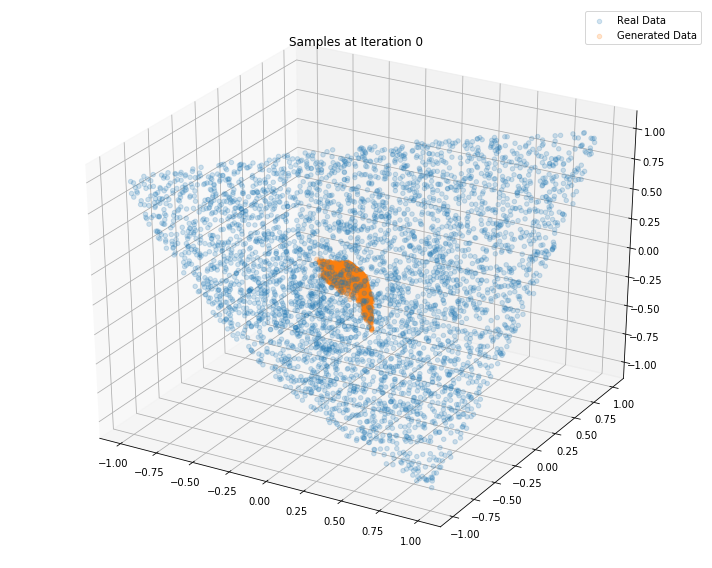``````Iterations: 100	 Discriminator loss: 1.3222	 Generator loss: 0.7560
``````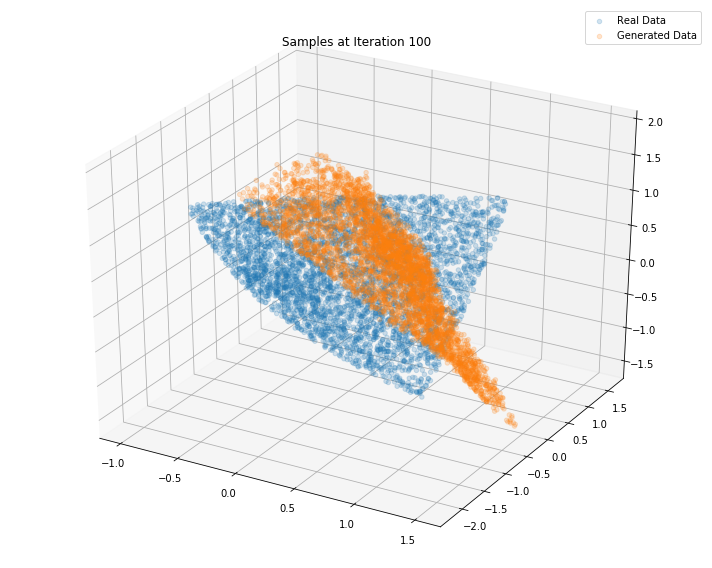``````Iterations: 200	 Discriminator loss: 1.4604	 Generator loss: 0.6441
````````````Iterations: 300	 Discriminator loss: 1.3710	 Generator loss: 0.8039
``````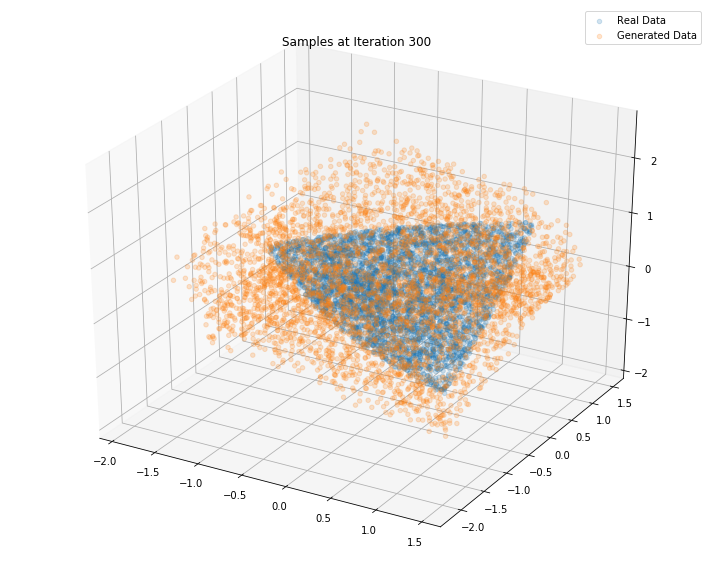``````Iterations: 400	 Discriminator loss: 1.3793	 Generator loss: 0.7000
````````````Iterations: 500	 Discriminator loss: 1.3865	 Generator loss: 0.6892
````````````Iterations: 600	 Discriminator loss: 1.3851	 Generator loss: 0.7015
``````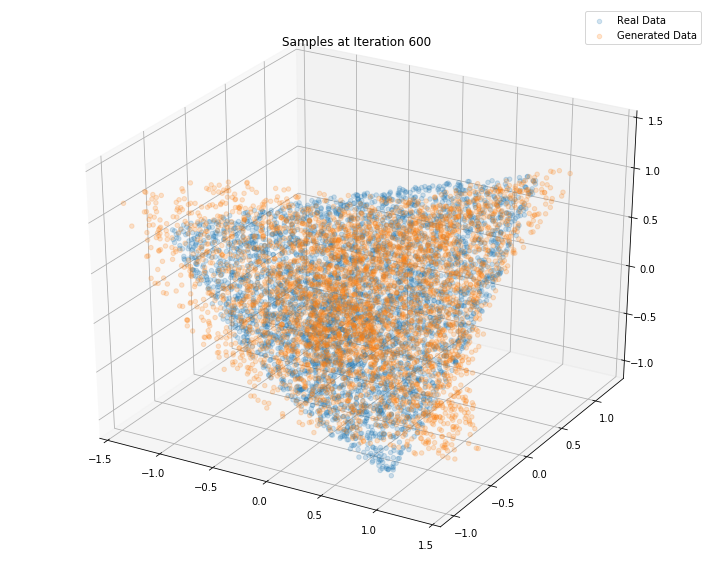``````Iterations: 700	 Discriminator loss: 1.3903	 Generator loss: 0.6932
``````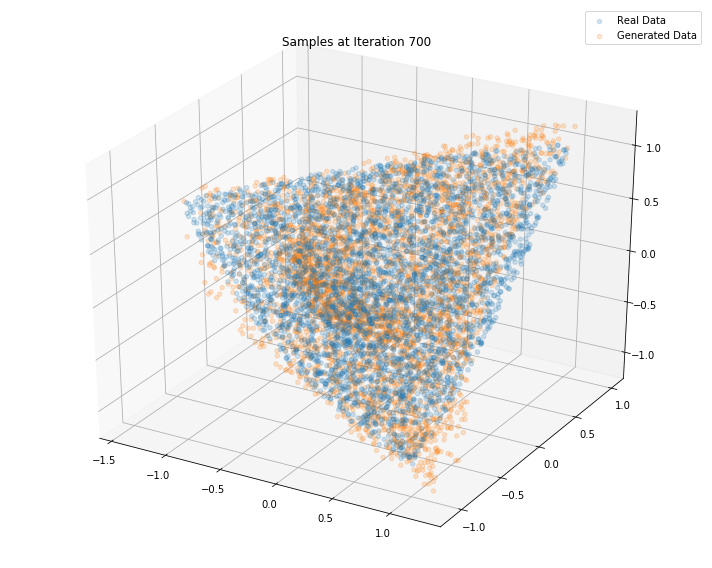``````Iterations: 800	 Discriminator loss: 1.3854	 Generator loss: 0.6958
``````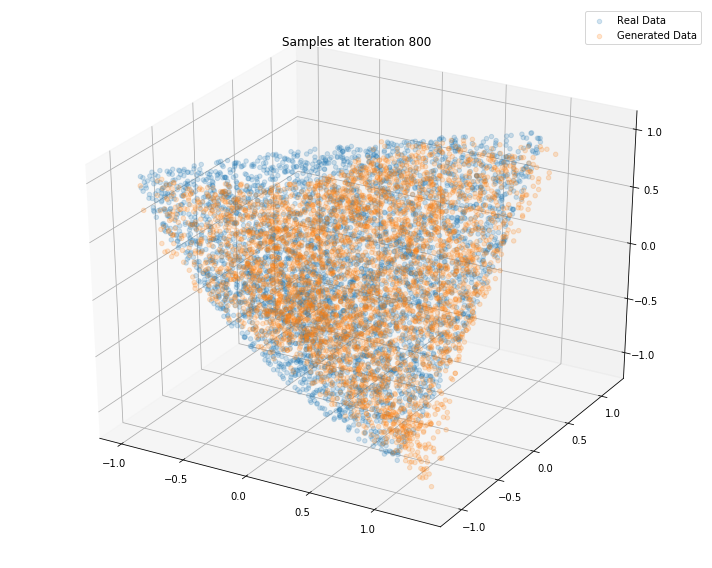``````Iterations: 900	 Discriminator loss: 1.3836	 Generator loss: 0.6837
````````````Iterations: 1000	 Discriminator loss: 1.3881	 Generator loss: 0.6930
````````````Iterations: 10000	 Discriminator loss: 1.3806	 Generator loss: 0.7006
``````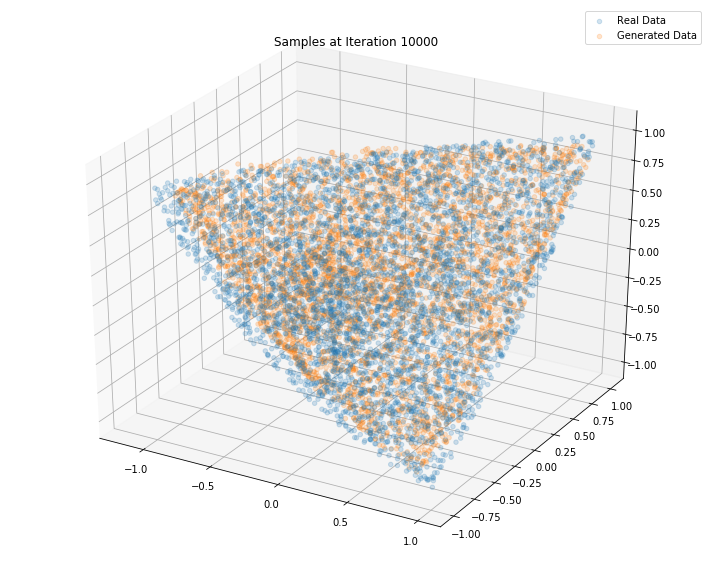``````Iterations: 20000	 Discriminator loss: 1.3824	 Generator loss: 0.6903
``````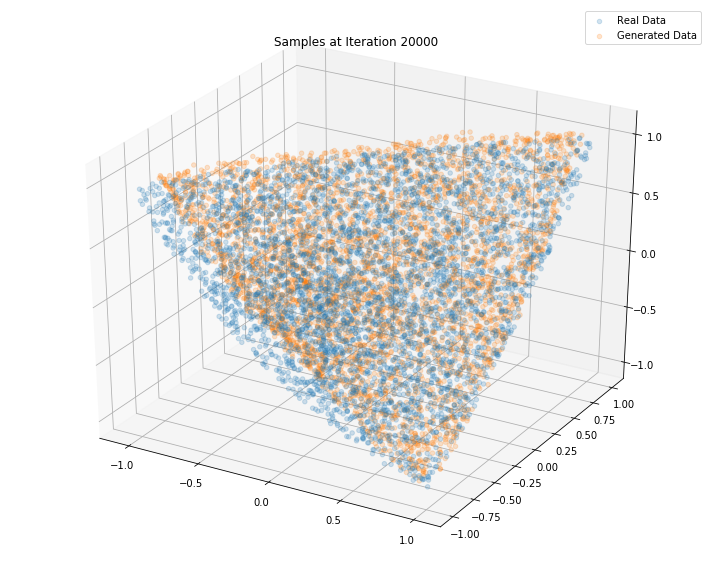``````Iterations: 30000	 Discriminator loss: 1.3694	 Generator loss: 0.6960
````````````Iterations: 40000	 Discriminator loss: 1.3579	 Generator loss: 0.6888
``````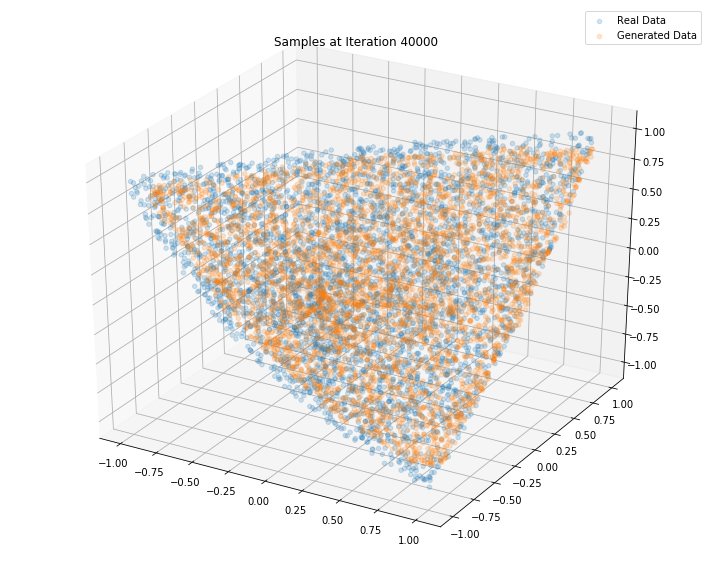``````Iterations: 50000	 Discriminator loss: 1.3454	 Generator loss: 0.6856
``````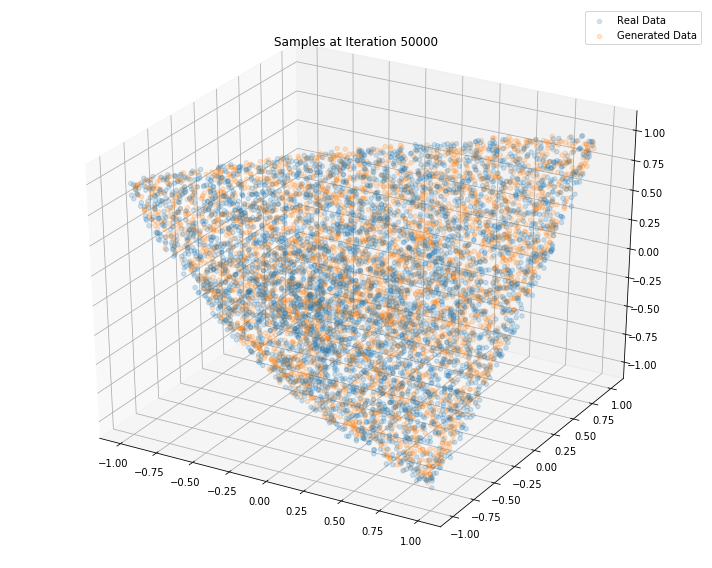``````Iterations: 60000	 Discriminator loss: 1.3551	 Generator loss: 0.7187
``````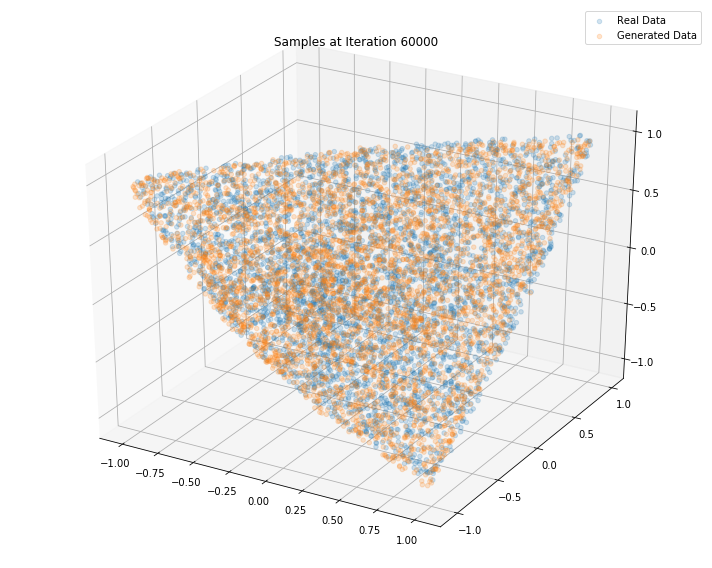``````Iterations: 70000	 Discriminator loss: 1.3141	 Generator loss: 0.7412
``````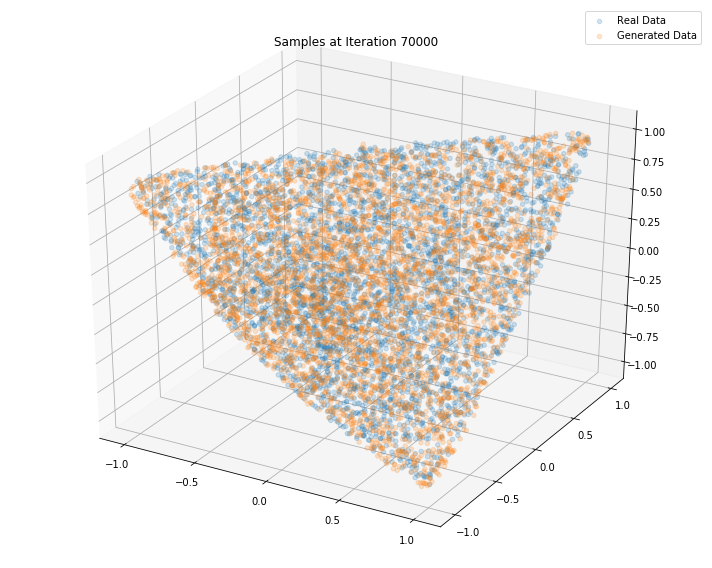``````Iterations: 80000	 Discriminator loss: 1.2773	 Generator loss: 0.7295
``````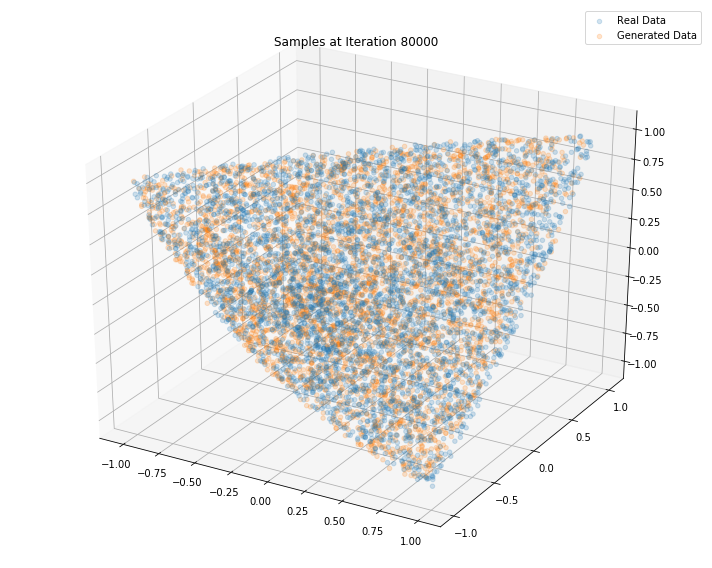``````Iterations: 90000	 Discriminator loss: 1.3026	 Generator loss: 0.7882
``````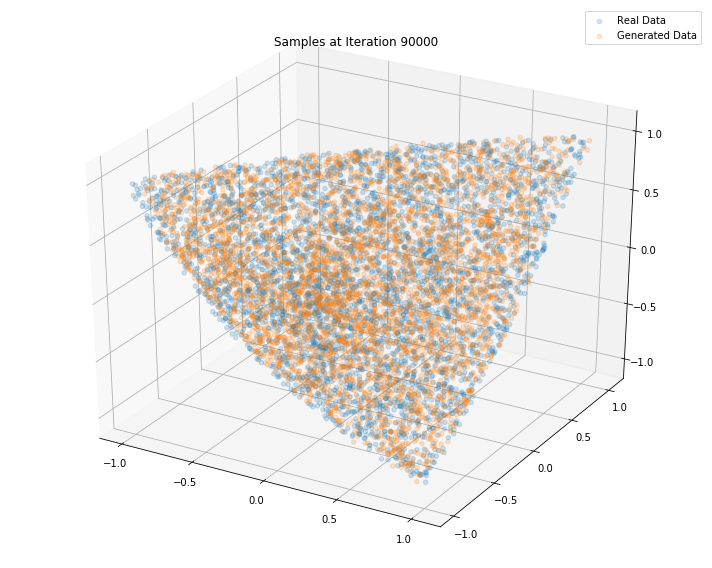``````n_dots = 2**14
x_plot = sample_data(n=n_dots)
Z_plot = sample_Z(n_dots, 3)

fig = plt.figure(figsize=(10, 8))
g_plot = sess.run(G_sample, feed_dict={Z: Z_plot})